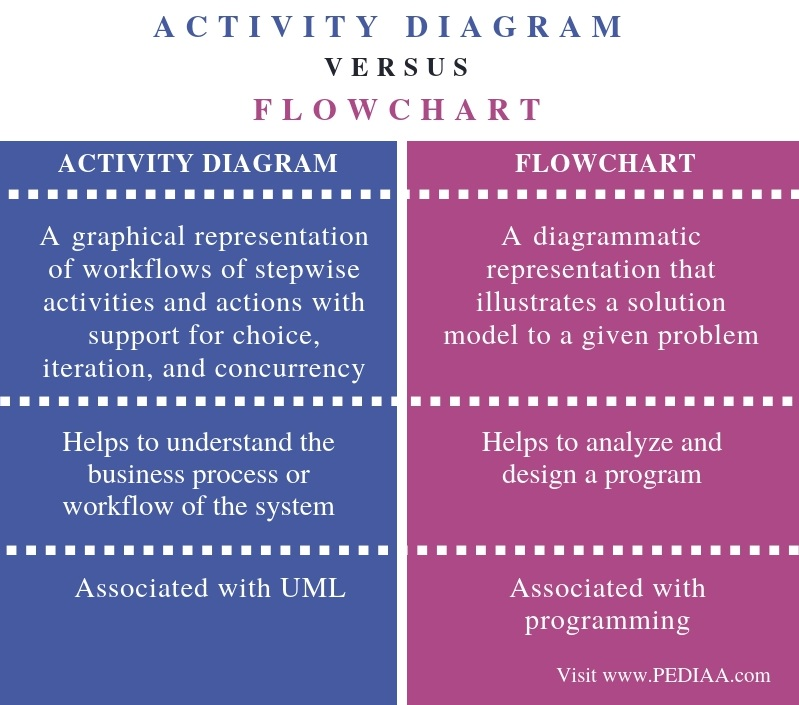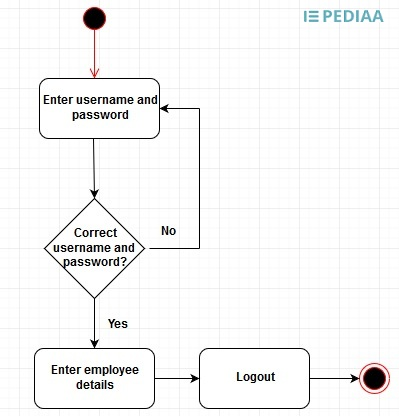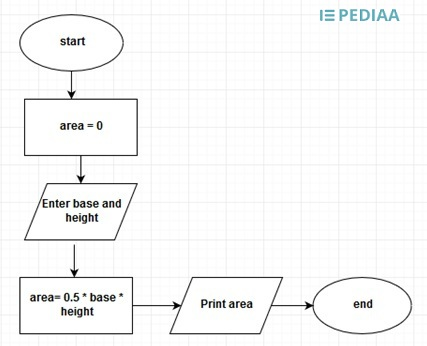# What is the Difference Between Activity Diagram and Flowchart

The main difference between activity diagram and flowchart is that an activity diagram is a UML behavior diagram that represents the workflow of stepwise activities of the system while a flowchart is a graphical diagram that represents the sequence of steps to solve a problem.

UML stands for Unified Modeling Language. It is different from other programming languages as it does not use any coding. Moreover, it is a visual modeling language to visualize, construct and document software. Most importantly, it provides a pictorial representation of the software. Therefore, any user can easily learn it. An activity diagram is a UML diagram. A flowchart, on the other hand, is a graphical diagram that represents an algorithm

### Key Areas Covered

1. What is an Activity Diagram
– Definition, Functionality
2. What is a Flowchart
– Definition, Functionality
3. What is the Difference Between Activity Diagram and Flowchart
– Comparison of Key Differences

### Key Terms

Activity Diagram, Algorithm, Flowchart## What is Activity Diagram

An activity diagram is a UML behavior diagram. It represents how each activity flows one after the other. An activity is some kind of an operation of the system. Furthermore, activity diagrams help business and development teams of an organization to understand the system processes and behaviors.Figure 1: Activity Diagram

Above is an activity diagram for a simple employee details entering system. The black circle represents the start node of the activity diagram. Each step is an action; the first step is to enter username and password. Moreover, the diamond symbol represents a decision node. If the username and password are correct, the user can enter employee details. If they are wrong, then the user has to enter the correct username and password. After entering the employee details, the user can log out. Finally, the end node represents the termination of the diagram.

## What is a Flowchart

An algorithm is a step by step procedure to solve a given problem. A flowchart is a diagrammatic representation of an algorithm. In computer programming, the flowchart diagram helps to write down an algorithm to solve the problem.Figure 2: Flowchart

The above flowchart calculates the area of a triangle. The oval symbol represents the start of the flowchart while the rectangle represents a process. The first rectangle is the process of initializing the variable area. The rhombus denotes an input or output operation. The input operating is to enter values for base and height. Next step is a process. It is the calculation of the area of the triangle. Next step is an output operation. Therefore, a rhombus represents printing the variable area. Finally, the oval symbol terminates the flowchart.  Throughout the activity diagram, the arrows represent the sequence of steps.

## Difference Between Activity Diagram and Flowchart

### Definition

An activity diagram is a graphical representation of workflows of stepwise activities and actions with support for choice, iteration, and concurrency. In contrast, a flowchart is a diagrammatic representation that illustrates a solution model to a given problem. Thus, this is the main difference between activity diagram and flowchart.

### Usage

Also, an activity diagram helps to understand the business process or workflow of the system while a flowchart helps to analyze and design a program.

### Association

Another difference between activity diagram and flowchart is that an activity diagram is associated with UML whereas a flowchart is associated with programming.

### Conclusion

The main difference between activity diagram and flowchart is that activity diagram is a UML behavior diagram that represents the workflow of stepwise activities of the system while flowchart is a graphical diagram that represents the sequence of steps to solve a problem. Even though both these have similar symbols, there is a distinct difference between activity diagram and flowchart.

##### Reference:

1. “UML Activity Diagram Tutorial.” Lucidchart, 6 Sept. 2018, Available here.
2. “What Is a Flowchart.” Lucidchart, 6 Sept. 2018, Available here.### About the Author: Lithmee

Lithmee holds a Bachelor of Science degree in Computer Systems Engineering and is reading for her Master’s degree in Computer Science. She is passionate about sharing her knowldge in the areas of programming, data science, and computer systems.